# Line Plots for Fractions

##By Icon Math

Make line plots to display measurements in fractions of a unit.# graphs and data line plot -"dot plots"

##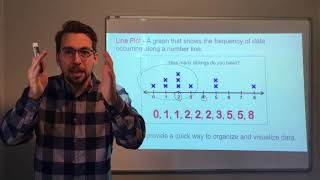By Joann's School

Learn how to read and create a Line Plot (Dot Plot)# Interpreting Line plots

##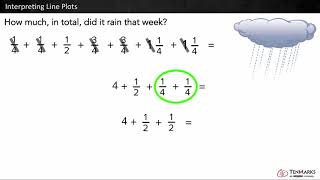By Tenmarks Amazon

Students learn to interpret line plots by using the given data to answer questions involving fractions and whole numbers.# Line Plots with Fractional Data

##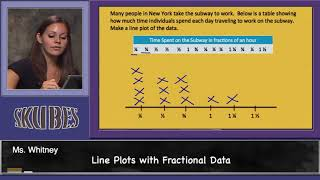By Skubes ed

Make a line plot to display a data set of measurements in fractions of a unit (1/2, 1/4, 1/8)# Introduction to line plots | Measurement and data | Early Math | Khan Academy

##By KhanAcademy##By KhanAcademy# Understanding Measurement Data Displayed in Line Plots: 2.MD.9

##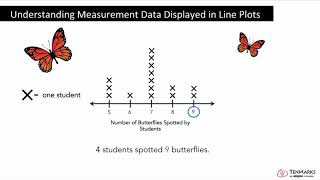By Tenmarks Amazon

Students learn how to interpret measurement data displayed in line plots.# [4.MD.4-1.0] Fractional Line Plots - Common Core Standard

##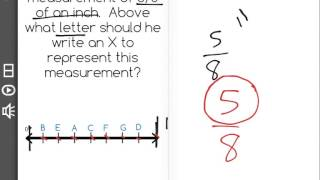By Freckle education

Make a line plot to display a data set of measurements in fractions of a unit (1/2, 1/4, 1/8)# Plot Fractions on the Number Line

##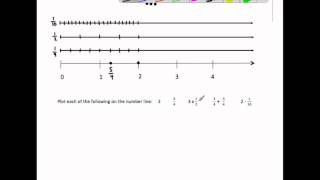By MathwithMrAlmeida

This video plots 6 fractions and operations with fractions on the number line. It ends by plotting all the same fractions on the number line and identify a pair of equivalent fractions and comparing each number's distance from zero.##By Escholarindia Faridabad# Plotting Fractions on a Number Line

##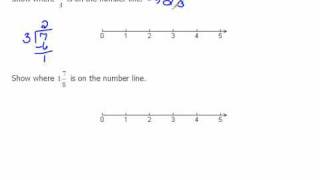By mathlablady4

The instructor first reviews how to plot whole numbers on a number line. She then relates the same concept to fractions. In the video lesson she plots both proper and improper fractions. Very clear instruction and easy for students to visualize the concept.# Line Plot Showing Measurement Data

##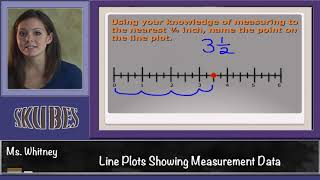By Skubes ed

Generate measurement data by measuring lengths using rulers marked with halves and fourths of an inch. Show the data by making a line plot, where the horizontal scale is marked off in appropriate units— whole numbers, halves, or quarters.# Line plots and stem-and-leaf plots

##By MathPlanetVideos

Construct a stem-and-leaf plot out of the following data: 6, 6,7,8,9,13,16,19,21,25,26# Fractions and Line Plots

##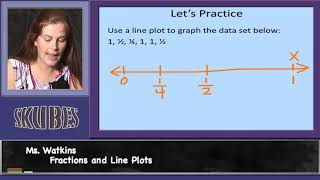By Skubes ed

Use the four operations to solve word problems involving distances, intervals of time, liquid volumes, masses of objects, and money, including problems involving simple fractions or decimals, and problems that require expressing measurements given in a larger unit in terms of a smaller unit.# Solving problems with line plots 1

##By Khan Academy

Create a line plot from a set of data. ï¿½ï¿½ï¿½ï¿½ï¿½ï¿½ï¿½ï¿½ï¿½ï¿½ï¿½ï¿½Use the line plot to answer questions.##By Khan Academy

Learn how to read a line plot that records average monthly rainfall for various cities.# line plot

##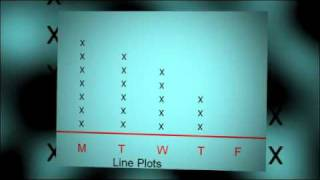By mrscornishmath# An introduction to geometry

##By MathPlanetVideos

Plot a pint, a line, a line segment and an angle in a coordinate plane.# Creating frequency tables

##By Khan Academy

In this video, we organize data into frequency tables and dot plots (sometimes called line plots).# Creating dot plots

##By Khan Academy

In this video, we organize data into frequency tables and dot plots (sometimes called line plots).